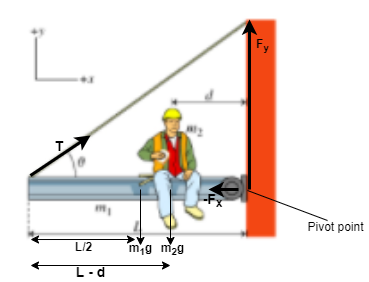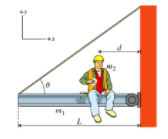# Problem: A uniform steel beam of length L and mass m1 is attached via a hinge to the side of a building. The beam is suspended by a steel cable attached to the end of the beam at an angle θ as shown. Through the hinge, the wall exerts an unknown force F on the beam. A workman of mass m2 sits eating lunch at a distance d from the building as shown in the figure. Part A. Find T, the tension in the cable. Remember to account for all the forces in the problem. Express your answer in terms of m1, m2, L, d, θ, and g, the magnitude of the acceleration due to gravity. Part B. Find Fx, the x-component of the force exerted by the wall on the beam ( F), using the axis shown. Remember to pay attention to the direction that the wall exerts the force. Express your answer in terms of T and other given quantities. Part C. Find Fy, the y-component of force that the wall exerts on the beam (F), using the axis shown. Remember to pay attention to the direction that the wall exerts the force. Express your answer in terms of T, θ, m1 , m2, and g

###### FREE Expert Solution

Let's visualize our problem in the figure below:85% (216 ratings)###### Problem Details

A uniform steel beam of length L and mass m1 is attached via a hinge to the side of a building. The beam is suspended by a steel cable attached to the end of the beam at an angle θ as shown. Through the hinge, the wall exerts an unknown force F on the beam. A workman of mass m2 sits eating lunch at a distance d from the building as shown in the figure.Part A. Find T, the tension in the cable. Remember to account for all the forces in the problem. Express your answer in terms of m1, m2, L, d, θ, and g, the magnitude of the acceleration due to gravity.

Part B. Find Fx, the x-component of the force exerted by the wall on the beam ( F), using the axis shown. Remember to pay attention to the direction that the wall exerts the force. Express your answer in terms of T and other given quantities.

Part C. Find Fy, the y-component of force that the wall exerts on the beam (F), using the axis shown. Remember to pay attention to the direction that the wall exerts the force. Express your answer in terms of T, θ, m1 , m2, and g